# Problem: Which of the following is the best Lewis symbol for bromine?A. B. C. D. E. Covalent bonds involve the sharing of valence electrons. Valence electrons are those in the outermost subhsells of an atom. Nitrogen, for example, has an electron configuration of 1s22s22p3. Therefore, nitrogen has five valence electrons (two in the 2s and three in the 2p). Lewis symbols or Lewis structures show all the valence electrons in an atom or in a molecule using dots, respectively. The Lewis symbol of nitrogen could be drawn like this:Five dots are drawn around N because nitrogen has five valence electrons. Also, since nitrogen must gain three electrons to achieve a noble-gas configuration, it will usually form three covalent bonds. That is why three of the dots are shown unpaired (ready for bonding) while the other two dots are shown paired (nonbonding).Fluorine, with electron configuration 1s22s22p5, has seven valence electrons (two in the 2s subshell and five in the 2p). Fluorine only needs to gain one electron to achieve a noble-gas configuration. Therefore, the Lewis symbol of F should only show one unpaired electron, while the rest should be paired:The Lewis structure (electron-dot structure) of the molecule NF3 is drawn as follows:Each bonding electron from nitrogen is paired with the bonding electron from a fluorine atom. Notice that each atom is now surrounded by eight dots, called an octet. An octet is desirable because it means that the outermost s and p subshells are full; in other words, they have a noble-gas configuration.

###### FREE Expert Solution
96% (339 ratings)View Complete Written Solution
###### Problem Details

Which of the following is the best Lewis symbol for bromine?

A.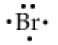B.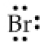C.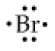D.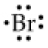E.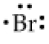Covalent bonds involve the sharing of valence electrons. Valence electrons are those in the outermost subhsells of an atom. Nitrogen, for example, has an electron configuration of 1s22s22p3. Therefore, nitrogen has five valence electrons (two in the 2s and three in the 2p). Lewis symbols or Lewis structures show all the valence electrons in an atom or in a molecule using dots, respectively. The Lewis symbol of nitrogen could be drawn like this: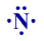Five dots are drawn around N because nitrogen has five valence electrons. Also, since nitrogen must gain three electrons to achieve a noble-gas configuration, it will usually form three covalent bonds. That is why three of the dots are shown unpaired (ready for bonding) while the other two dots are shown paired (nonbonding).

Fluorine, with electron configuration 1s22s22p5, has seven valence electrons (two in the 2s subshell and five in the 2p). Fluorine only needs to gain one electron to achieve a noble-gas configuration. Therefore, the Lewis symbol of F should only show one unpaired electron, while the rest should be paired: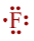The Lewis structure (electron-dot structure) of the molecule NF3 is drawn as follows: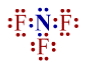Each bonding electron from nitrogen is paired with the bonding electron from a fluorine atom. Notice that each atom is now surrounded by eight dots, called an octet. An octet is desirable because it means that the outermost s and p subshells are full; in other words, they have a noble-gas configuration.